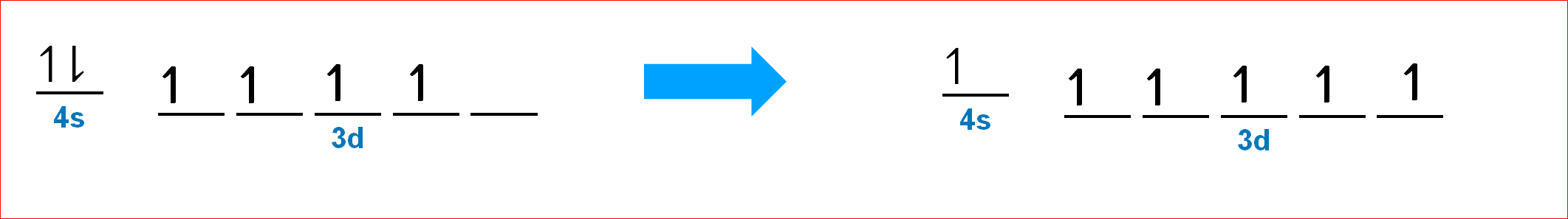# Problem: The total number of electrons in the 3d orbitals of Cr3+ isa. 1.b. 2.c. 3.d. 4.e. 5.

###### FREE Expert Solution

Neutral Cr: (transition metal)

Atomic number → 24 → 24 e-

Recall:

s           _                1 orbital   →  max of 2 electrons

p        _ _ _             3 orbitals  → max of 6 electrons

d       _ _ _ _ _        5 orbitals → max of 10 electrons

Fill up the orbitals with electrons:

Aufbau Principle  totally fill in the lowest energy orbital first
Hund’s Rule
electron orbitals that are degenerate (same energy) are first half-filled before they are totally filled

Electron configuration (lowest energy level to the highest energy level: s < p < d < f):

• n = energy level → row/period number
• Cr: 1s22s22p63s23p64s23d4
• But, d orbitals half filled or fully filledexception• Ground state electron configuration:
• Cr: [Ar]3d5 or 1s22s22p63s23p64s13d5###### Problem Details

The total number of electrons in the 3d orbitals of Cr3+ is

a. 1.

b. 2.

c. 3.

d. 4.

e. 5.